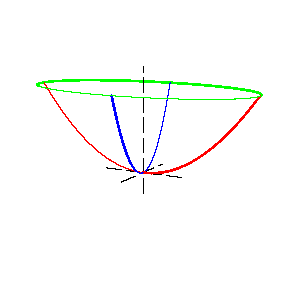# Elliptic paraboloid

(diff) ← Older revision | Latest revision (diff) | Newer revision → (diff)
Jump to: navigation, search

A non-closed surface of the second order. The canonical equation of an elliptic paraboloid has the form

$$\frac{x^2}{p}+\frac{y^2}{q}=2z,\quad p,q>0.$$Figure: e035510a

In this form, an elliptic paraboloid is situated on one side of the $Oxy$-plane (see Fig.). The sections by planes parallel to the $Oxy$-plane are ellipses with equal eccentricity. (If $p=q$ they are circles, and the surface is called a paraboloid of revolution.) The sections by planes passing through the $Oz$-axis are parabolas. The sections by the planes $Oyz$ and $Oxz$ are called the principal parabolas. The axis of symmetry of an elliptic paraboloid is called its axis and the point of intersection of the axis with the elliptic paraboloid is its vertex.

How to Cite This Entry:
Elliptic paraboloid. Encyclopedia of Mathematics. URL: http://www.encyclopediaofmath.org/index.php?title=Elliptic_paraboloid&oldid=31978
This article was adapted from an original article by A.B. Ivanov (originator), which appeared in Encyclopedia of Mathematics - ISBN 1402006098. See original article